SSS

Chapter 7 Class 9 Triangles
Concept wise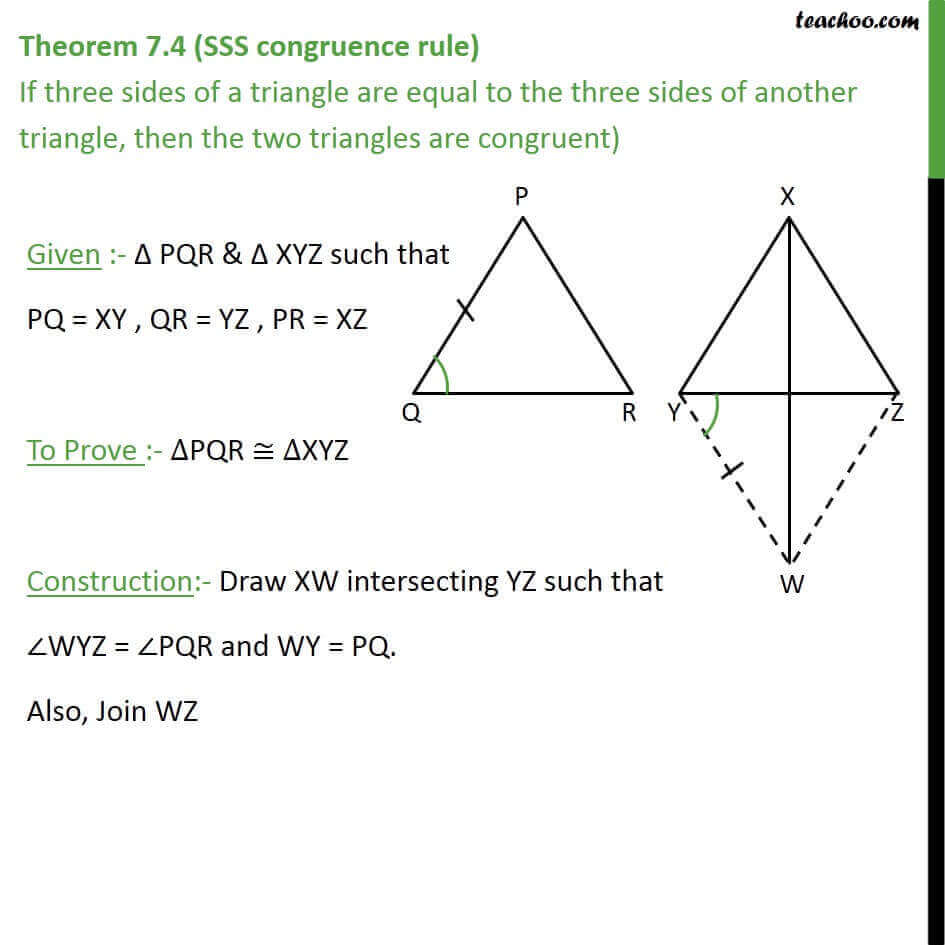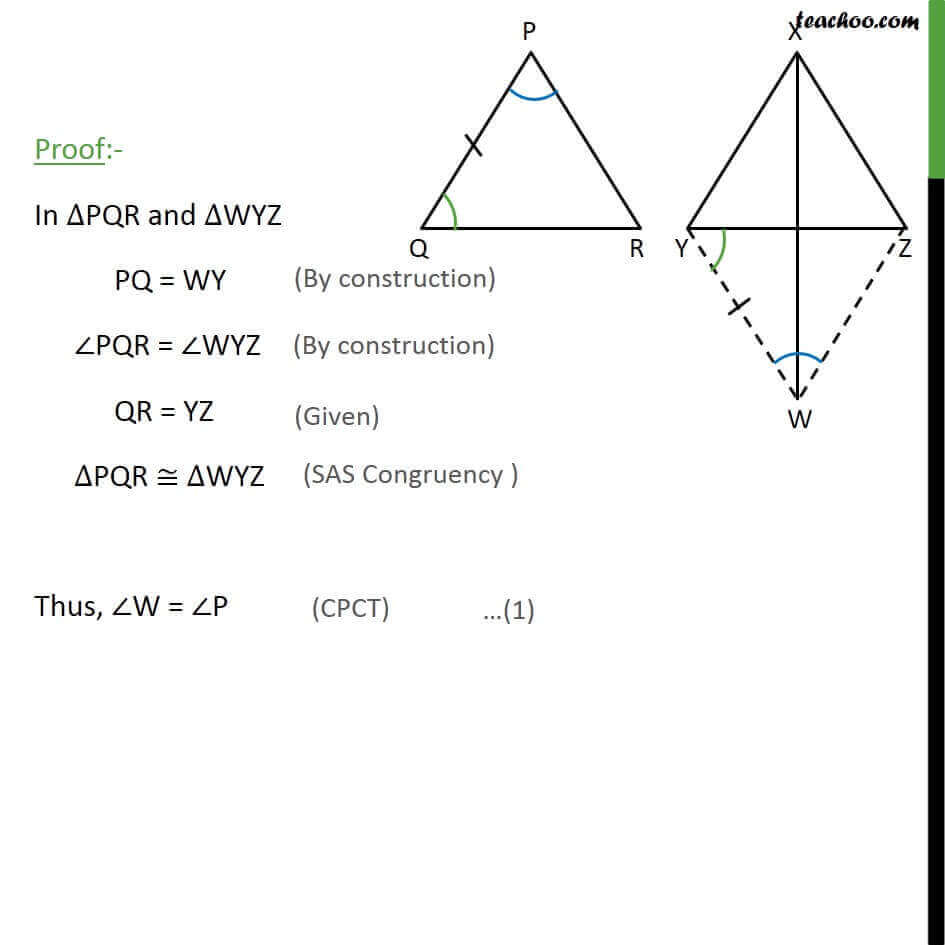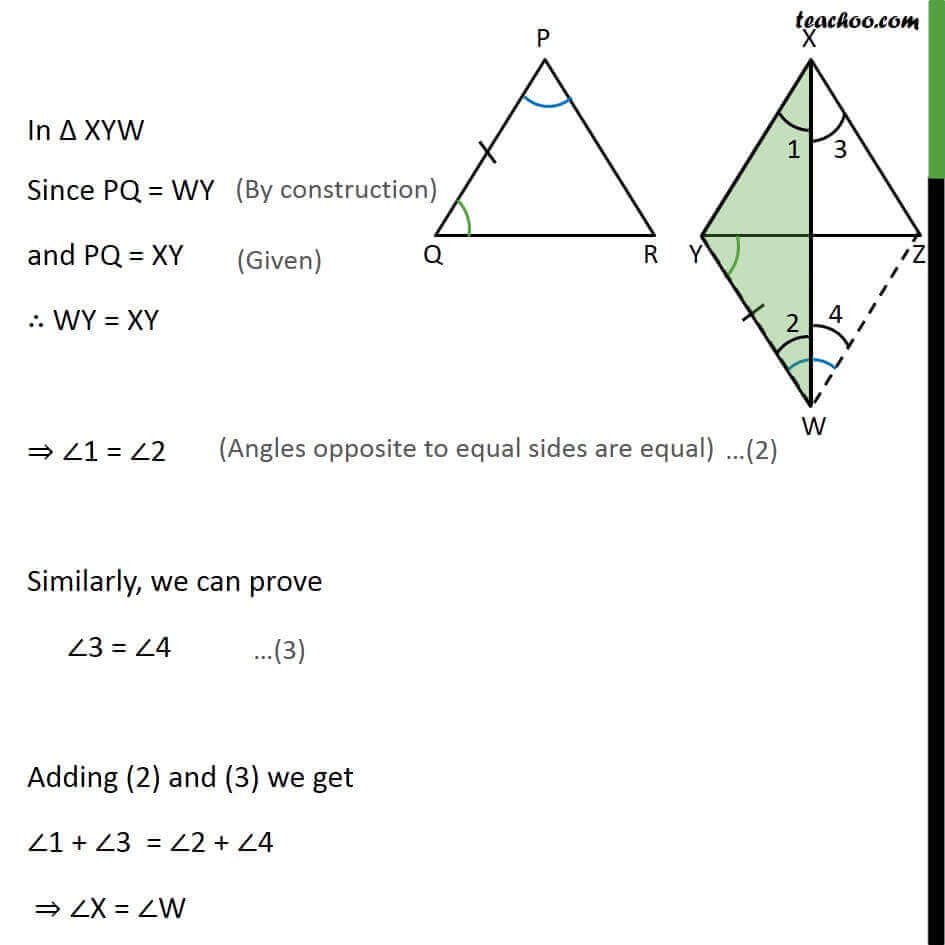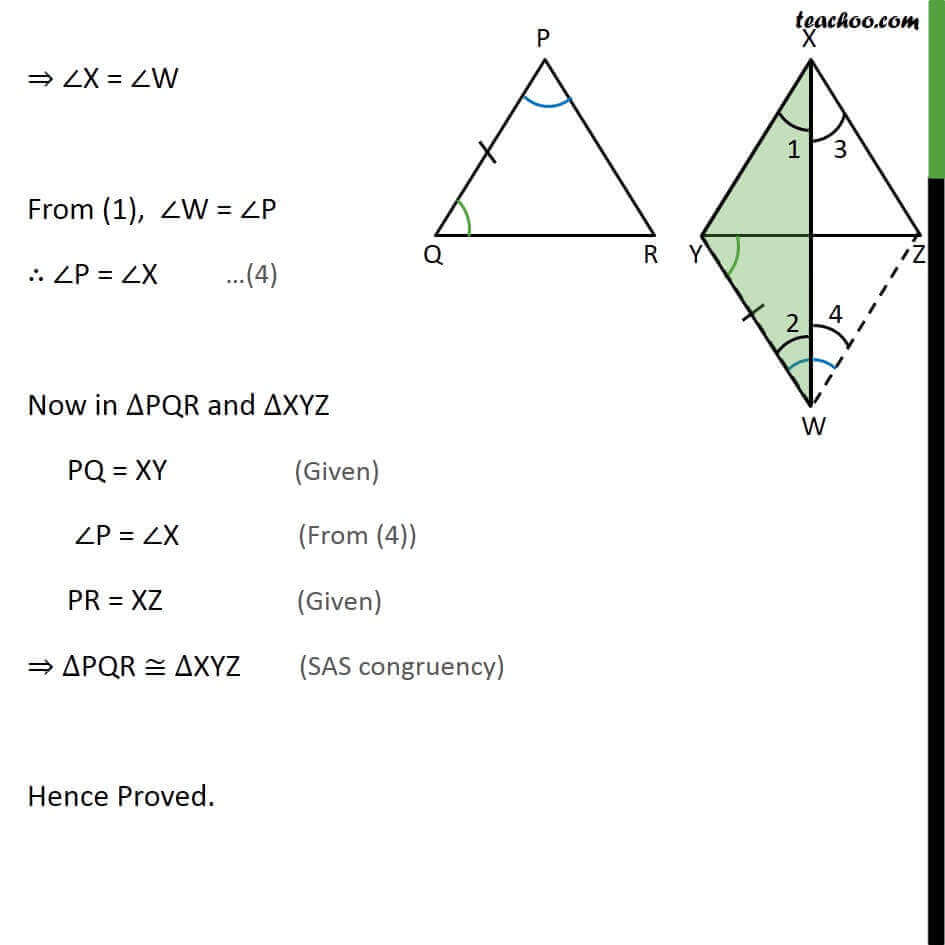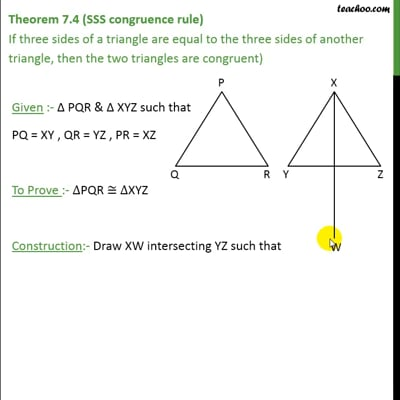This video is only available for Teachoo black users

Introducing your new favourite teacher - Teachoo Black, at only ₹83 per month

### Transcript

Theorem 7.4 (SSS congruence rule) If three sides of a triangle are equal to the three sides of another triangle, then the two triangles are congruent) Given :- PQR & XYZ such that PQ = XY , QR = YZ , PR = XZ To Prove :- PQR XYZ Construction:- Draw XW intersecting YZ such that WYZ = PQR and WY = PQ. Also, Join WZ Proof:- In PQR and WYZ PQ = WY PQR = WYZ QR = YZ PQR WYZ Thus, W = P In XYW Since PQ = WY and PQ = XY WY = XY 1 = 2 Similarly, we can prove 3 = 4 Adding (2) and (3) we get 1 + 3 = 2 + 4 X = W X = W From (1), W = P P = X Now in PQR and XYZ PQ = XY P = X PR = XZ PQR WYZ Hence Proved.